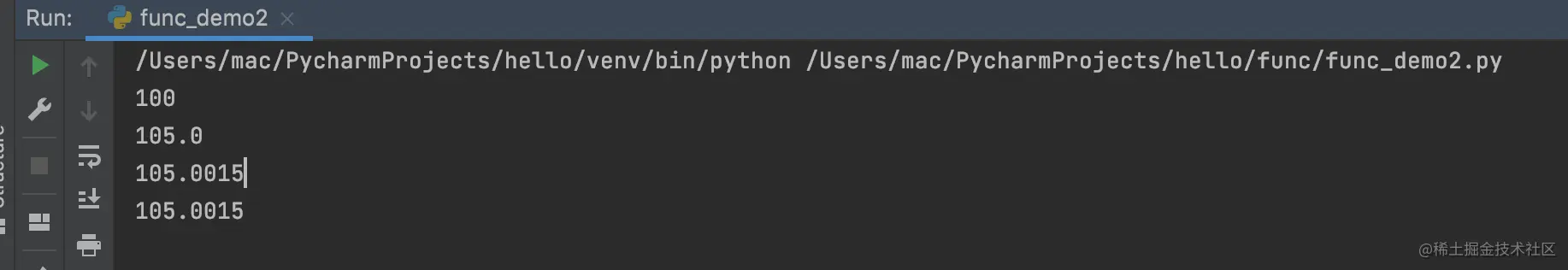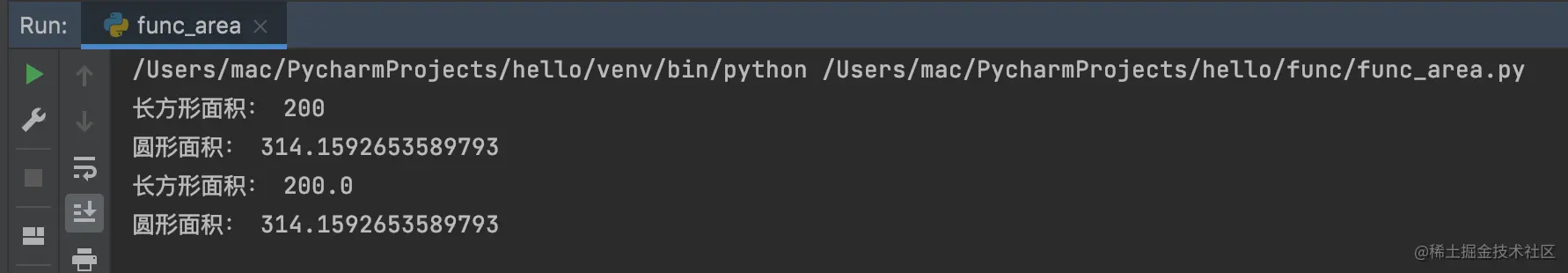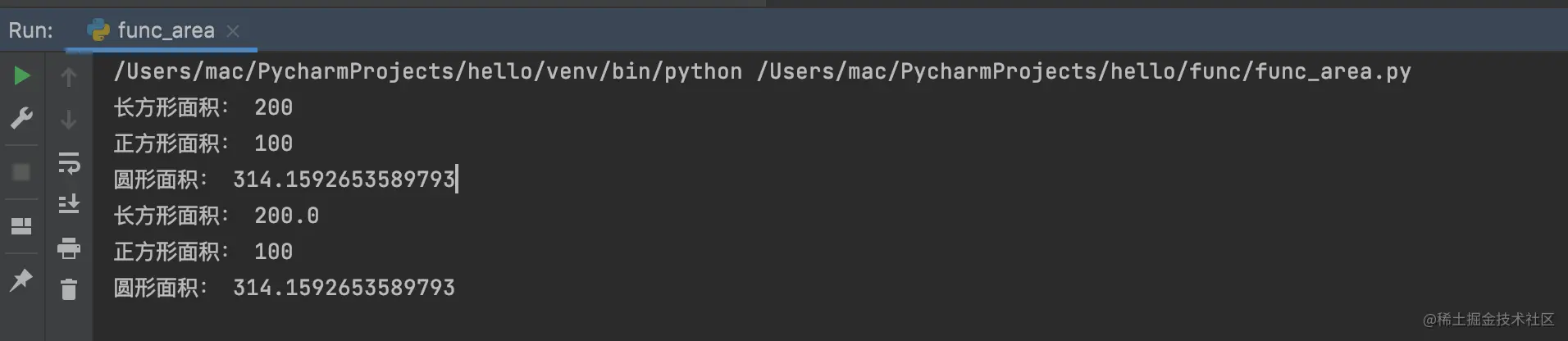# Talk about ordinary functions and higher-order functions in Python

2022-01-30 19:25:24

Little knowledge , Great challenge ！ This article is participating in 「 A programmer must have a little knowledge 」 Creative activities

This article has participated in  「 Digging force Star Program 」 , Win a creative gift bag , Challenge creation incentive fund .

ceremonial Python Column No 25 piece , Classmate, stop , Don't miss this from 0 The beginning of the article ！

Today, the school committee is writing code , Wrote a lot of , Let's talk again this time python The function in

# What is a function

Every language has functions , Even people use Excel There are also functions , We used to learn many kinds of functions in Mathematics .

Python The same goes for functions in .

def f(x):
print(" Parameter is ：",x)
return x
Copy code 

Functions here y = f(x), In mathematics, it is expressed as a slope of 1 The straight line of .

# Nested calls to functions

def z(x):
pass

def f(x):
print(" Parameter is ：",x)
return z(x)
Copy code 

like this , We are f(x) Called in z（x） function （ It's used here pass keyword , Implementation without writing , For display purposes only ）

Can we not define z(x) Just define a function and call other functions ？

It's like realizing the square of a number , Functional ‘ square ’, About that .

## Higher order function

def f(z):
return z()
Copy code 

This is the higher order function ,f The function needs an external parameter , This parameter must be a function .

In the use of f(z) When , We can't give one f(2), f(3) So the value of the . Or there's a function like d(x) Returns a result that is not a function value , We can't call... Like this :f（d(1)).

The school committee has prepared the following code , From a simple function to a higher-order function ：

#!/usr/bin/env python
# -*- coding: utf-8 -*-
# @Time : 2021/10/24 11:39  Afternoon
# @Author : LeiXueWei
# @CSDN/Juejin/Wechat:  Lei Xuewei
# @XueWeiTag: CodingDemo
# @File : func_demo2.py
# @Project : hello

def f1(x):
return x

def f2(x, z=100):
return x + z / 10

def f3(x, z=100, *dynamic_args):
sum = 0
for arg in dynamic_args:
sum += arg
return x + z / 10 + sum / 10000.0

def dummy_sum(*args):
return 0

def f4(x, z=100, sum_func=dummy_sum):
return x + z / 10 + sum_func() / 10000.0

print(f1(100))
print(f2(100, z=50))
print(f3(100, 50, 4, 5, 6))

def sum_g(*dynamic_args):
def sum_func():
sum = 0
for arg in dynamic_args:
sum += arg
return sum
return sum_func

print(f4(100, 50, sum_g(4, 5, 6)))
Copy code 

Here we see the function f1, f2, f3, f4.

Add a point of knowledge ： *dynamic_args It's a dynamic parameter , Parameters of indefinite length .
That is to say f3 Clearly stated 3 Parameters , Finally, we gave 5 Parameters .
here f3 Think x=100, z=50, dynamic_args = [4, 5, 6]

Let's look at the output first ：f3 and f4 It looks the same .

But the nature is completely different , The reader can think for ten seconds .

f4 Very elastic , Because the third parameter is .

Higher order functions can help us calculate ‘ Dimension reduction ’（ Three dimensional becomes two-dimensional , Two to one ）.

# Let's think about calculating the area of a circle and a square

I believe you can write the following two functions with your eyes closed ：

#!/usr/bin/env python
# -*- coding: utf-8 -*-
# @Time : 2021/10/24 11:39  Afternoon
# @Author : LeiXueWei
# @CSDN/Juejin/Wechat:  Lei Xuewei
# @XueWeiTag: CodingDemo
# @File : func_demo2.py
# @Project : hello
import math

def circle_area(r):
return math.pi * r * r

def rectangle_area(a, b):
return a * b
Copy code 

This is the mathematical formula for the circular area ：

$f(r) = \pi * r^2$

This is the mathematical formula of rectangular area ：

$f(a, b) = a * b$

We see that there are 1 Parameters , Some have two ways to become higher-order functions ？

Readers can think for a while .

Here is the code ：

#!/usr/bin/env python
# -*- coding: utf-8 -*-
# @Time : 2021/10/24 11:39  Afternoon
# @Author : LeiXueWei
# @CSDN/Juejin/Wechat:  Lei Xuewei
# @XueWeiTag: CodingDemo
# @File : func_demo2.py
# @Project : hello
import math

def circle_area(r):
return math.pi * r * r

def rectangle_area(a, b):
return a * b

def area(x, linear, factor):
return x * linear(x, factor)

def relation(x, factor):
return x * factor

a = 10
b = 20

print(" Rectangular area ：", rectangle_area(a, b))
print(" Circular area ：", circle_area(a))
print(" Rectangular area ：", area(a, relation, factor=b / a))
print(" Circular area ：", area(a, relation, factor=math.pi))
Copy code 

The results are as follows ：This is just a solution .

You can see that from the code , We regard both a circle and a rectangle as a reference （ radius / One side ） The square of , Multiply by a factor .

below , Let's add... To the square area calculation ：

#!/usr/bin/env python
# -*- coding: utf-8 -*-
# @Time : 2021/10/24 11:39  Afternoon
# @Author : LeiXueWei
# @CSDN/Juejin/Wechat:  Lei Xuewei
# @XueWeiTag: CodingDemo
# @File : func_demo2.py
# @Project : hello
import math

def circle_area(r):
return math.pi * r * r

def square_area(a):
return a * a

def rectangle_area(a, b):
return a * b

def area(x, linear, factor):
return x * linear(x, factor)

def relation(x, factor):
return x * factor

a = 10
b = 20

print(" Rectangular area ：", rectangle_area(a, b))
print(" Square area ：", square_area(a))
print(" Circular area ：", circle_area(a))
print(" Rectangular area ：", area(a, relation, factor=b / a))
print(" Square area ：", area(a, relation, factor=1))
print(" Circular area ：", area(a, relation, factor=math.pi))
Copy code 

The result of the above code execution is as follows ：This is the magic of higher-order functions , Let's think from the perspective of a square .

Just one area Functions and relation function , Neither of these functions need to be modified , Just give one factor（ Empirical factor ）, You can quickly calculate its area .

## Why higher-order functions can reduce the dimension

A function of the area calculated from the distance above , We can see how to calculate circles and rectangles , Can be regarded as a one-dimensional function .

Then take the square area as the reference , Quickly estimate the area of circles and squares .

Of course, the radius is used to calculate the circular area above , It's not intuitive enough , Readers can change it to diameter , such factor = math.pi / 4.

This will be more appropriate in feeling .

# summary

In addition to the functions described above , Parameters , Higher order function . We can also use lambda function ：

lambda   Parameters 1,  Parameters 2,..., The first n Parameters  ：  Calculation expression
Copy code 

The function above relation Functions can be omitted without writing , Finally, the call is changed to ：

print(" Rectangular area ：", area(a, lambda x, f: x * f, factor=b / a))
print(" Square area ：", area(a, lambda x, f: x * f, factor=1))
print(" Circular area ：", area(a, lambda x, f: x * f, factor=math.pi))
Copy code 

by the way , like Python Friend, , Please pay attention to Python Basic column or Python From getting started to mastering the big column

Continuous learning and continuous development , I'm Lei Xuewei ！
Programming is fun , The key is to understand the technology thoroughly .
Welcome to wechat , Like support collection !